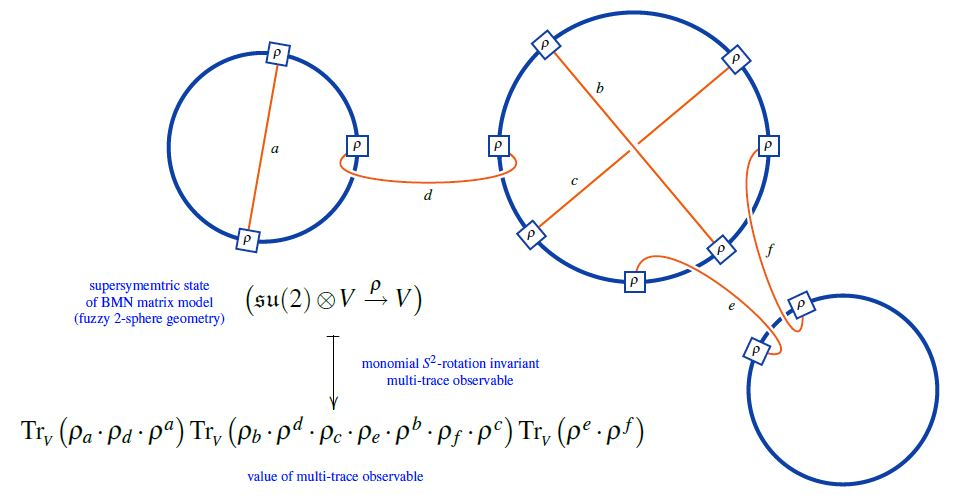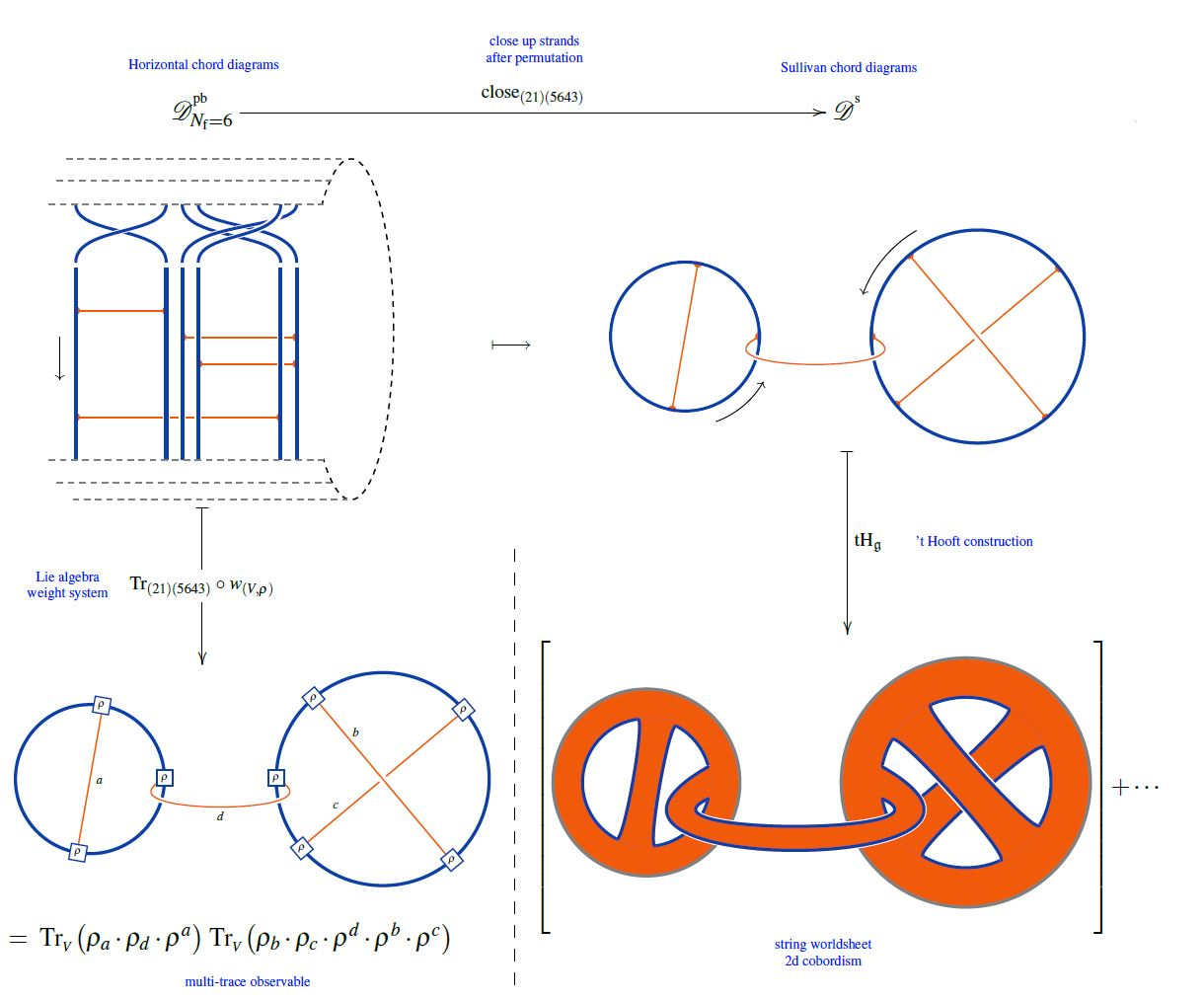Contents

# Contents

## Idea

A multi trace observable in a gauge theory is a polynomial in single trace operators (see there for more background).

## Properties

Under the AdS/CFT correspondence, single trace observables in the gauge theory correspond to single particle/string excitations on the gravity-side, while multi-trace observables correspond to multi-particle/string excitations (Liu 98, p. 6 (7 of 39), Andrianapoli-Ferrara 99, p. 13, Chalmers-Schalm 00, Section 7, Aharony-Gubser-Maldacena-Ooguri-Oz 99, p. 75)

The asymptotic boundary conditions for fields $\Phi$ on the AdS-side

$\Phi(\vec x, r) \overset{r \to 0} \sum_i \alpha_i (\vec x) r^{d -\lambda_i} + \cdots$

that correspond to multi-trace observables $W(\{\mathcal{O}_i\})$ have coefficients given by the derivative of the multi-trace polynomial by its single-trace variables $\mathcal{O}_i$:

$\alpha_i = \partial_{\mathcal{O}_i} W (\{\langle\mathcal{O}_i\rangle\})$

## Examples

### Chord diagrams as multi-trace observables in the BMN matrix model

The supersymmetric states of the BMN matrix model are temporally constant complex matrices which are complex metric Lie representations $\mathfrak{g} \otimes V \overset{\rho}{\to} V$ of $\mathfrak{g}=$su(2) (interpreted as fuzzy 2-sphere noncommutative geometries of giant gravitons or equivalently as fuzzy funnels of D0-D2 brane bound states).

A fuzzy 2-sphere-rotation invariant multi-trace observable on these supersymmetric states is hence an expression of the following form:(from Sati-Schreiber 19c)

Here we are showing the corresponding string diagram/Penrose notation for metric Lie representations, which makes manifest that

1. these multi-trace observables are encoded by Sullivan chord diagrams $D$

2. their value on the supersymmetric states $\mathfrak{su}(2) \otimes V \overset{\rho}{\to}V$ is the evaluation of the corresponding Lie algebra weight system $w_{{}_V}$ on $D$.

Or equivalently, if $\widehat D$ is a horizontal chord diagram whose $\sigma$-permuted closure is $D$ (see here) then the values of the invariant multi-trace observables on the supersymmetric states of the BMN matrix model are the evaluation of $w_{V,\sigma}$ on $\widehat D$, as shown here:(from Sati-Schreiber 19c)

But since all horizontal weight systems are partitioned Lie algebra weight systems this way, this identifies supersymmetric states of the BMN matrix model as seen by invariant multi-trace observables as horizontal chord diagrams evaluated in Lie algebra weight systems.

Discussion of multi-trace operators in super Yang-Mills theory and of their AdS-CFT dual gravity/string theory incarnation:

Textbook account: